# How to use the SUMPRODUCT function in Excel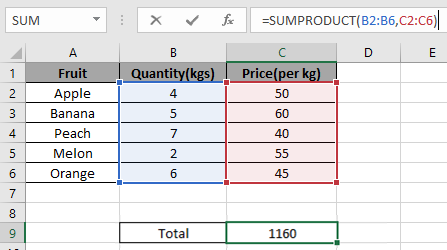In this article, we will learn How to use the SUMPRODUCT function in Excel.

Calculate the Total price multiplying quantity with Price per product plus Interest and other ?

Mostly working at grocery shopping stores where you need to multiply some values (quantity, price, tax) for each product and then take the SUM of all the products to get the total order price.

For example we have 9 values. Here we need to multiply in each row and then sum the column (where the multiplied result is stored).

 3 7 6 126 5 9 2 90 1 4 8 32 248

Total= ( 3 * 7 * 6 + 5 * 9 * 2 + 1 * 4 * 8 ) = 248

This is hectic when you just need the total price for the order. Excel provides you with the SUMPRODUCT function which does this task. Let's learn how to use SUMPRODUCT function.

SUMPRODUCT Function in Excel

SUMPRODUCT function is a mathematical function in Excel. It operates on multiple ranges. It multiplies the corresponding arrays and then adds them.

Syntax :

 =SUMPRODUCT (array1, [array2], ...)

First array : first array as reference. Like A1:A10 (10 values)

Second array : second array as reference. Like A1:A10 (10 values)

Thirst array, fourth array, fifth goes on.

Example :

All of these might be confusing to understand. Let's understand how to use the function using an example. Here we have a vegetable store and the job is to calculate the bill for individual customers.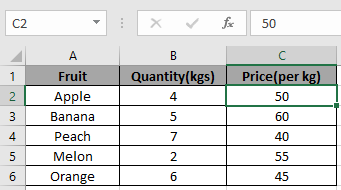We need to find the total amount for the order.

Use the formula

 =SUMPRODUCT(B2:B6,C2:C6)

You will get the total here.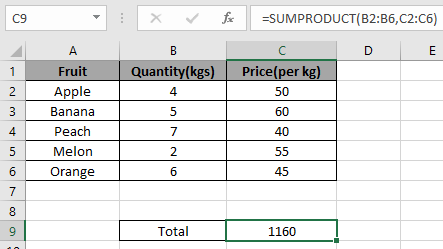If there’s text in any cell will be considered as 0.

SUMPRODUCT function can be used in counting cells having condition or criteria.

Another example

So, let's test this formula via running it on the example shown below.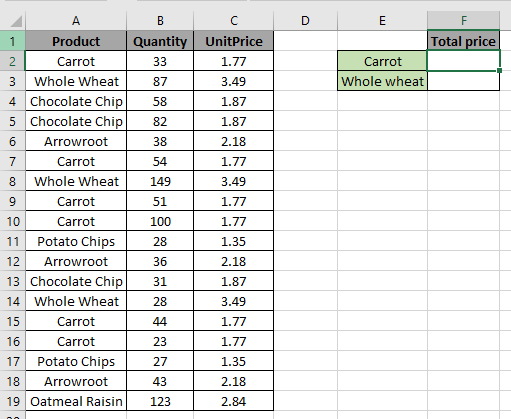Here the problem is we need to find the total price for the product “Carrot”.

So here we use the formula to get the total price for Carrot

 =SUMPRODUCT(--(A2:A19="Carrot"), B2:B19, C2:C19)

A2:A19 : checks the range A2:A19 for the text “Carrot” and returns True if finds “Carrot” or else False

-- : the operator decodes True as 1 and False as 0.

B2:B19 : Quantity (range) to be multiplied with Unit Price.

C2:C19 : Unit price (range) to be multiplied with Quantity.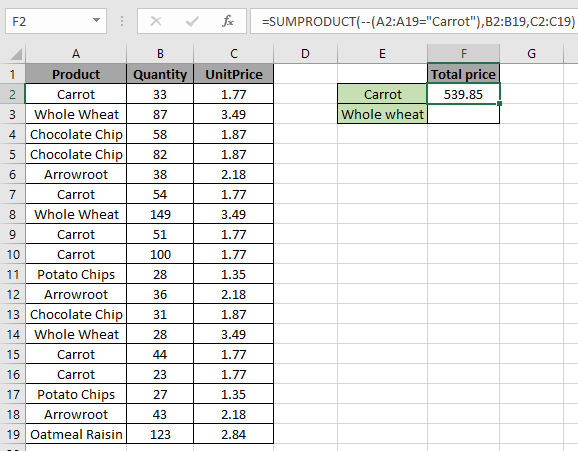Product Quantity UnitPrice Price 1 33 1.77 58.41 0 87 3.49 0 0 58 1.87 0 0 82 1.87 0 0 38 2.18 0 1 54 1.77 95.58 0 149 3.49 0 1 51 1.77 90.27 1 100 1.77 177 0 28 1.35 0 0 36 2.18 0 0 31 1.87 0 0 28 3.49 0 1 44 1.77 77.88 1 23 1.77 40.71 0 27 1.35 0 0 43 2.18 0 0 123 2.84 0 539.85

As you have understood from the above snapshot and table, that SUMPRODUCT function multiplies corresponding values and returns the Sum as outcome.

1’s & 0’s in the left most column is the IF logic used in SUMPRODUCT function.

So here we use the formula for total price for Whole wheat

 =SUMPRODUCT(--(A2:A19="Whole wheat"),B2:B19,C2:C19)

A2:A19: checks the range A2:A19 for the text “Whole wheat” and returns True if finds “Carrot” or else False

-- : the operator decode True as 1 and False as 0.

B2:B19 : Quantity (range) to be multiplied with Unit Price.

C2:C19 : Unit price (range) to be multiplied with Quantity.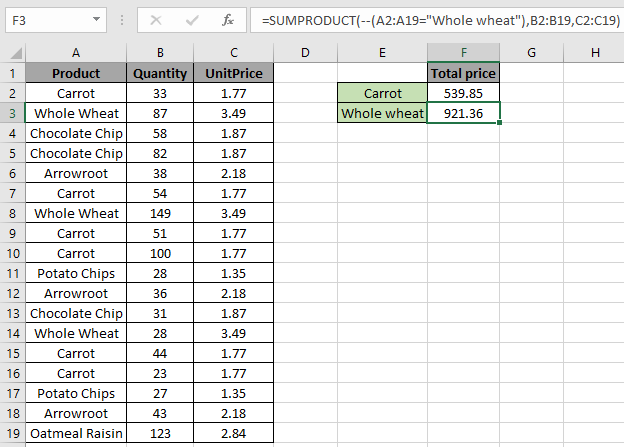Product Quantity UnitPrice Price 0 33 1.77 0 1 87 3.49 303.63 0 58 1.87 0 0 82 1.87 0 0 38 2.18 0 0 54 1.77 0 1 149 3.49 520.01 0 51 1.77 0 0 100 1.77 0 0 28 1.35 0 0 36 2.18 0 0 31 1.87 0 1 28 3.49 97.72 0 44 1.77 0 0 23 1.77 0 0 27 1.35 0 0 43 2.18 0 0 123 2.84 0 921.36

As you have understood from the above snapshot and table, that SUMPRODUCT function multiplies corresponding values and returns the Sum.

1’s & 0’s in the left most column is the IF logic used with SUMPRODUCT function.

Here are all the observational notes using the SUMPRODUCT function in Excel
Notes :

1. The formula only works with only to look up the exact match.
2. The SUMPRODUCT function considers non-numeric values as 0s.
3. The SUMPRODUCT function considers logic value TRUE as 1 and False as 0.
4. The argument array must be the same size else the function returns an error.
5. SUMPRODUCT function returns the value corresponding to the TRUE values in the returned array after taking individual products in the corresponding array.
6. Operators like equals to ( = ), less than equal to ( <= ), greater than ( > ) or not equals to ( <> ) can be performed within a formula applied, with numbers only.

Hope this article about How to use the SUMPRODUCT function in Excel is explanatory. Find more articles on calculating values and related Excel formulas here. If you liked our blogs, share it with your friends on Facebook. And also you can follow us on Twitter and Facebook. We would love to hear from you, do let us know how we can improve, complement or innovate our work and make it better for you. Write to us at info@exceltip.com.

Related Articles :

Sum by OFFSET groups in Rows and Columns : The OFFSET function can be used to sum group of cells dynamically. These groups can be anywhere in the sheet.

SUM if date is between : Returns the SUM of values between given dates or period in excel.

Sum if date is greater than given date: Returns the SUM of values after the given date or period in excel.

2 Ways to Sum by Month in Excel: Returns the SUM of values within a given specific month in excel.

How to Sum Multiple Columns with Condition: Returns the SUM of values across multiple columns having condition in excel

Use INDEX and MATCH to Lookup Value : The INDEX-MATCH formula is used to lookup dynamically and precisely a value in a given table. This is an alternative to the VLOOKUP function and it overcomes the shortcomings of the VLOOKUP function.

How to use wildcards in excel : Count cells matching phrases using the wildcards in excel.

Popular Articles :

How to use the IF Function in Excel : The IF statement in Excel checks the condition and returns a specific value if the condition is TRUE or returns another specific value if FALSE.

How to use the VLOOKUP Function in Excel : This is one of the most used and popular functions of excel that is used to lookup value from different ranges and sheets.

How to use the SUMIF Function in Excel : This is another dashboard essential function. This helps you sum up values on specific conditions.

How to use the COUNTIF Function in Excel : Count values with conditions using this amazing function. You don't need to filter your data to count specific values. Countif function is essential to prepare your dashboard.

Terms and Conditions of use

The applications/code on this site are distributed as is and without warranties or liability. In no event shall the owner of the copyrights, or the authors of the applications/code be liable for any loss of profit, any problems or any damage resulting from the use or evaluation of the applications/code.